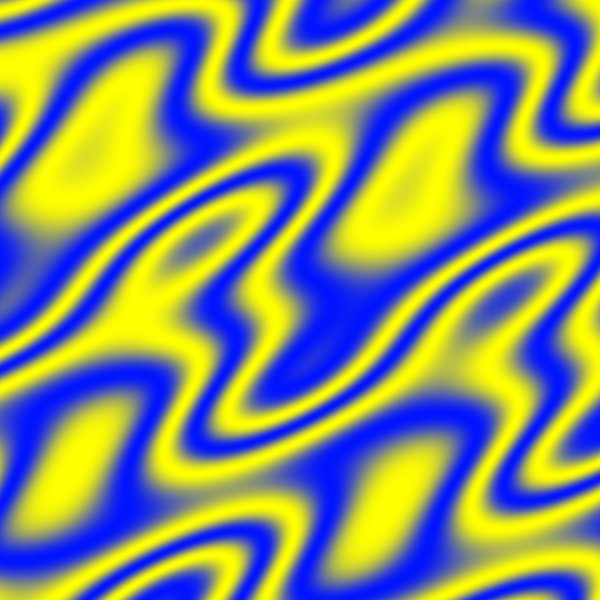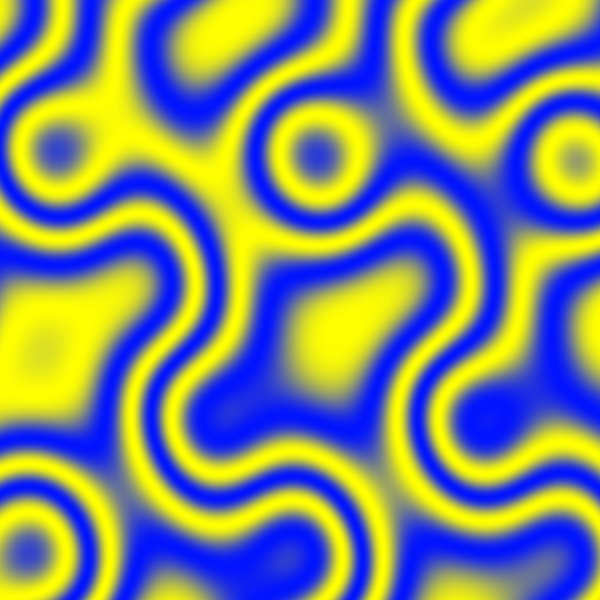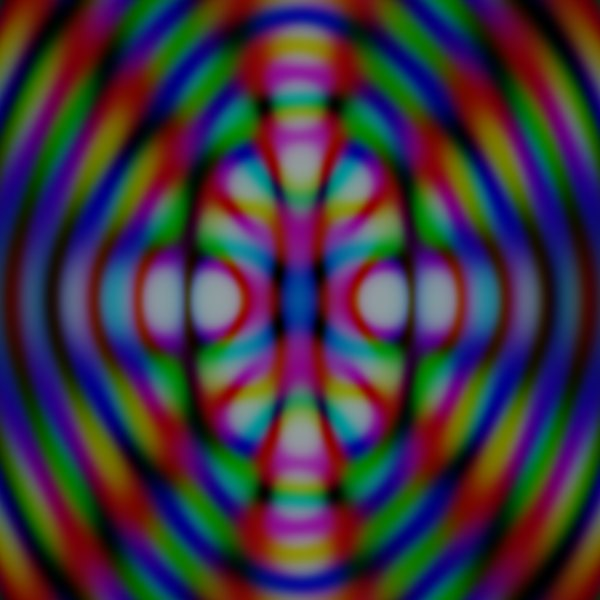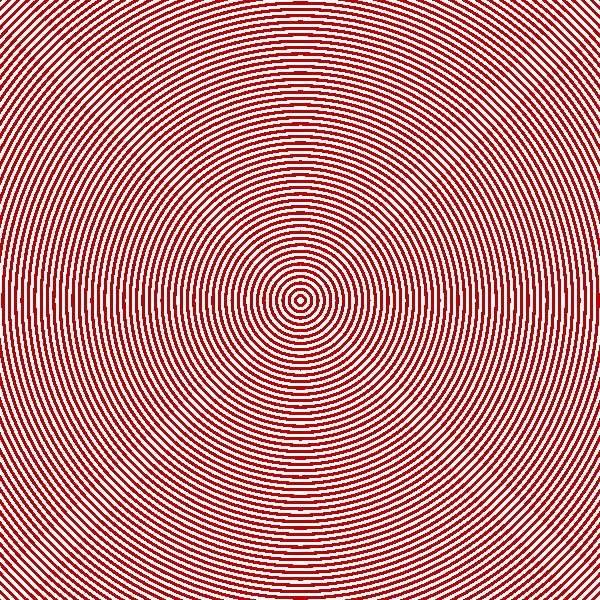Code and Stuff

## Nov 29, 2011

### HTML5 Canvas Image composition

A view on the composition of two images at changing position can result in quite interesting effects. Especially if we use the correct images and composition function.## The Code

The implementation is quite simple. In the initialization javascript function we will load the two images and trigger the drawing.
```var images = [];
function init() {
images = new Image();
images.src = "URL to first image";
images = new Image();
images.src = "URL to second image";
window.setTimeout("draw()", 50);
}
```

The draw function will simply place the two images at different positions. The `globalCompositeOperation` attribute sets the composition type.
```var t = 0;
function draw() {
var canvas = document.getElementById("ID of canvas");
var ctx = canvas.getContext("2d");

ctx.globalCompositeOperation = 'copy'; // forget the past
// draw first image
ctx.drawImage(images, 0, 0);

var px = -150.0 + 150.0 * Math.sin(0.86 * t + 2.3) * Math.sin(13 + t * 0.52);
var py = -200.0 + 200.0 * Math.sin(t * 1.3)*  Math.sin(13 + t * 0.32);

ctx.globalCompositeOperation = 'lighter';
ctx.drawImage(images, px, py);

// increase the iteration
t = t + 0.06;

// reschedule
window.setTimeout("draw()", 50);
}
```
Note: the position of the first image can also be changed to alter the behaviour (as in the examples below).

On the HTML part we simply have to place the canvas and have someone call the initialization function.

```<canvas width="300" height="200" id="canvas_ID"></canvas>
// For simplicity we us a link to start the animation
<a onclick="init()" href="#">Start</a>
```

## Other examples

The choice of input images, how we move them and the composition function will greatly affect the result.

The effect works also when the two images are the same. Still adding composition with an image of circles.Concentric circles and using xor composition.## Nov 16, 2011

### Problem

Call an overloaded `operator=` on a temporary instance of a class.

Given a class `A`:

```struct A {
A(int arg) { }
A& operator=(int num) { return *this; }
};
```
The following code will not compile with gcc-4.6.1 due to a "conflicting declaration" error:
```int a;
A(a) = 4; // error: conflicting declaration
```
The compiler thinks that with `A(a)` we are trying to declare an instance of `A` named `a`.

### Workaround

Wrapping the instantiation with round brackets will fix the problem.
```int a;
(A(a)) = 4;
```

Strangely, when the operator is not the assignment one, the code compiles.

```struct A {
A(int arg) { }
A& operator+=(int num) { return *this; }
};
...
int a;
A(a) += 4;
```

## Nov 10, 2011

### Single class

Given a class `A` with a default constructor, the following code:
`A a();`
is a declaration of a function that returns an object of type `A` and takes no arguments. You will get a compilation error when you try to call a method on `a` or pass it to some other method/function. To create an instance of `A` you have to avoid the brackets and simply use
`A a;`

### 2 classes

In addition to the simple class `A`, lets define also a class `B` as follows:
```struct B {
B(const A& a) {}
};
```

Just as in the example above,

```B b1(A());
B b2(A);
```
declares 2 functions. The first returns an instance of `B` and takes, as parameter, a function with no inputs and which returns `A`. The second also returns `B`, but has a parameter of type `A`.

To create an instance of `B` with a temporary default constructed instance of `A` you will have to wrap the creation of the temporary instance with round brackets.

```B b1((A()));
```